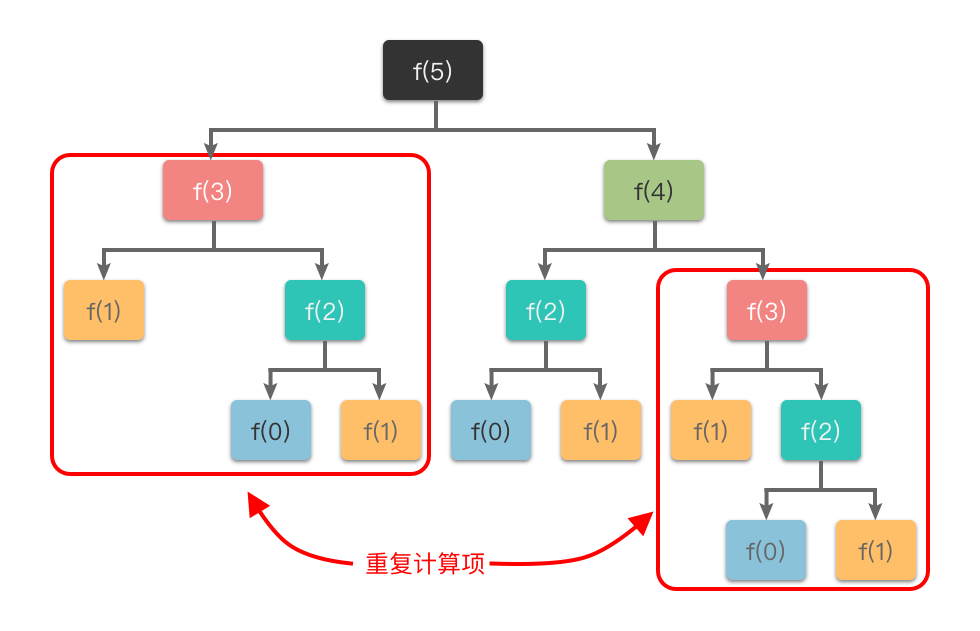01.动态规划基础知识

# 动态规划基础知识 #

## 1. 动态规划简介 #

### 1.2 动态规划的核心思想 #

1. 把「原问题」分解为「若干个重叠的子问题」，每个子问题的求解过程都构成一个 「阶段」。在完成一个阶段的计算之后，动态规划方法才会执行下一个阶段的计算。
2. 在求解子问题的过程中，按照「自顶向下的记忆化搜索方法」或者「自底向上的递推方法」求解出「子问题的解」，把结果存储在表格中，当需要再次求解此子问题时，直接从表格中查询该子问题的解，从而避免了大量的重复计算。

1. 适用于动态规划求解的问题，在分解之后得到的子问题往往是相互联系的，会出现若干个重叠子问题。
2. 使用动态规划方法会将这些重叠子问题的解保存到表格里，供随后的计算查询使用，从而避免大量的重复计算。

### 1.3 动态规划的简单例子 #

$f(n) = \begin{cases} 0 & n = 0 \cr 1 & n = 1 \cr f(n - 2) + f(n - 1) & n > 1 \end{cases}$1. 定义一个数组 $dp$，用于记录斐波那契数列中的值。
2. 初始化 $dp = 0，dp = 1$。
3. 根据斐波那契数列的递推公式 $f(n) = f(n - 1) + f(n - 2)$，从 $dp(2)$ 开始递推计算斐波那契数列的每个数，直到计算出 $dp(n)$。
4. 最后返回 $dp(n)$ 即可得到第 $n$ 项斐波那契数。

  1 2 3 4 5 6 7 8 9 10 11 12 13 14 15  class Solution: def fib(self, n: int) -> int: if n == 0: return 0 if n == 1: return 1 dp = [0 for _ in range(n + 1)] dp = 0 dp = 1 for i in range(2, n + 1): dp[i] = dp[i - 2] + dp[i - 1] return dp[n] 

## 2. 动态规划的特征 #

1. 最优子结构性质
2. 重叠子问题性质
3. 无后效性

### 2.1 最优子结构性质 #### 2.2 重叠子问题性质 #### 2.3 无后效性 ### 3. 动态规划的基本思路 #1. 划分阶段：将原问题按顺序（时间顺序、空间顺序或其他顺序）分解为若干个相互联系的「阶段」。划分后的阶段⼀定是有序或可排序的，否则问题⽆法求解。
• 这里的「阶段」指的是⼦问题的求解过程。每个⼦问题的求解过程都构成⼀个「阶段」，在完成前⼀阶段的求解后才会进⾏后⼀阶段的求解。
2. 定义状态：将和子问题相关的某些变量（位置、数量、体积、空间等等）作为一个「状态」表示出来。状态的选择要满⾜⽆后效性。
• 一个「状态」对应一个或多个子问题，所谓某个「状态」下的值，指的就是这个「状态」所对应的子问题的解。
3. 状态转移：根据「上一阶段的状态」和「该状态下所能做出的决策」，推导出「下一阶段的状态」。或者说根据相邻两个阶段各个状态之间的关系，确定决策，然后推导出状态间的相互转移方式（即「状态转移方程」）。
4. 初始条件和边界条件：根据问题描述、状态定义和状态转移方程，确定初始条件和边界条件。
5. 最终结果：确定问题的求解目标，然后按照一定顺序求解每一个阶段的问题。最后根据状态转移方程的递推结果，确定最终结果。

## 4. 动态规划的应用 #

### 4.1 斐波那契数 #

#### 4.1.2 题目大意 #

• 斐波那契数列的定义如下：
• $f(0) = 0, f(1) = 1$。
• $f(n) = f(n - 1) + f(n - 2)$，其中 $n > 1$。
• $0 \le n \le 30$。

• 示例 1：
 1 2 3  输入：n = 2 输出：1 解释：F(2) = F(1) + F(0) = 1 + 0 = 1 
• 示例 2：
 1 2 3  输入：n = 3 输出：2 解释：F(3) = F(2) + F(1) = 1 + 1 = 2 

#### 4.1.4 代码 #

  1 2 3 4 5 6 7 8 9 10 11 12  class Solution: def fib(self, n: int) -> int: if n <= 1: return n dp = [0 for _ in range(n + 1)] dp = 0 dp = 1 for i in range(2, n + 1): dp[i] = dp[i - 2] + dp[i - 1] return dp[n] 

#### 4.1.5 复杂度分析 #

• 时间复杂度：$O(n)$。一重循环遍历的时间复杂度为 $O(n)$。
• 空间复杂度：$O(n)$。用到了一维数组保存状态，所以总体空间复杂度为 $O(n)$。

### 4.2 爬楼梯 #

#### 4.2.2 题目大意 #

• $1 \le n \le 45$。

• 示例 1：
 1 2 3 4 5  输入：n = 2 输出：2 解释：有两种方法可以爬到楼顶。 1. 1 阶 + 1 阶 2. 2 阶 
• 示例 2：
 1 2 3 4 5 6  输入：n = 3 输出：3 解释：有三种方法可以爬到楼顶。 1. 1 阶 + 1 阶 + 1 阶 2. 1 阶 + 2 阶 3. 2 阶 + 1 阶 

#### 4.2.3 解题思路 #

###### 4. 初始条件 #
• 第 $0$ 层台阶方案数：可以看做 $1$ 种方法（从 $0$ 阶向上爬 $0$ 阶），即 $dp = 1$。
• 第 $1$ 层台阶方案数：$1$ 种方法（从 $0$ 阶向上爬 $1$ 阶），即 $dp = 1$。
• 第 $2$ 层台阶方案数：$2$ 中方法（从 $0$ 阶向上爬 $2$ 阶，或者从 $1$ 阶向上爬 $1$ 阶）。

#### 4.2.4 代码 #

 1 2 3 4 5 6 7 8 9  class Solution: def climbStairs(self, n: int) -> int: dp = [0 for _ in range(n + 1)] dp = 1 dp = 1 for i in range(2, n + 1): dp[i] = dp[i - 1] + dp[i - 2] return dp[n] 

#### 4.2.5 复杂度分析 #

• 时间复杂度：$O(n)$。一重循环遍历的时间复杂度为 $O(n)$。
• 空间复杂度：$O(n)$。用到了一维数组保存状态，所以总体空间复杂度为 $O(n)$。因为 $dp[i]$ 的状态只依赖于 $dp[i - 1]$ 和 $dp[i - 2]$，所以可以使用 $3$ 个变量来分别表示 $dp[i]$、$dp[i - 1]$、$dp[i - 2]$，从而将空间复杂度优化到 $O(1)$。

### 4.3 不同路径 #

#### 4.3.2 题目大意 #

• $1 \le m, n \le 100$。
• 题目数据保证答案小于等于 $2 * 10^9$。

• 示例 1：
 1 2  输入：m = 3, n = 7 输出：28 
• 示例 2：
 1 2 3 4 5 6 7  输入：m = 3, n = 2 输出：3 解释： 从左上角开始，总共有 3 条路径可以到达右下角。 1. 向右 -> 向下 -> 向下 2. 向下 -> 向下 -> 向右 3. 向下 -> 向右 -> 向下#### 4.3.3 解题思路 #

###### 4. 初始条件 #
• 从左上角走到 $(0, 0)$ 只有一种方法，即 $dp = 1$。
• 第一行元素只有一条路径（即只能通过前一个元素向右走得到），所以 $dp[j] = 1$。
• 同理，第一列元素只有一条路径（即只能通过前一个元素向下走得到），所以 $dp[i] = 1$。

#### 4.3.4 代码 #

  1 2 3 4 5 6 7 8 9 10 11 12 13 14  class Solution: def uniquePaths(self, m: int, n: int) -> int: dp = [[0 for _ in range(n)] for _ in range(m)] for j in range(n): dp[j] = 1 for i in range(m): dp[i] = 1 for i in range(1, m): for j in range(1, n): dp[i][j] = dp[i - 1][j] + dp[i][j - 1] return dp[m - 1][n - 1] 

#### 4.3.5 复杂度分析 #

• 时间复杂度：$O(m * n)$。初始条件赋值的时间复杂度为 $O(m + n)$，两重循环遍历的时间复杂度为 $O(m * n)$，所以总体时间复杂度为 $O(m * n)$。
• 空间复杂度：$O(m * n)$。用到了二维数组保存状态，所以总体空间复杂度为 $O(m * n)$。因为 $dp[i][j]$ 的状态只依赖于上方值 $dp[i - 1][j]$ 和左侧值 $dp[i][j - 1]$，而我们在进行遍历时的顺序刚好是从上至下、从左到右。所以我们可以使用长度为 $m$ 的一维数组来保存状态，从而将空间复杂度优化到 $O(m)$。